# Ackermann’s function using Dynamic programming

Given two non-zero integers M and N, the problem is to compute the result of the Ackermann function based on some particular equations.

Ackermann function is defined as: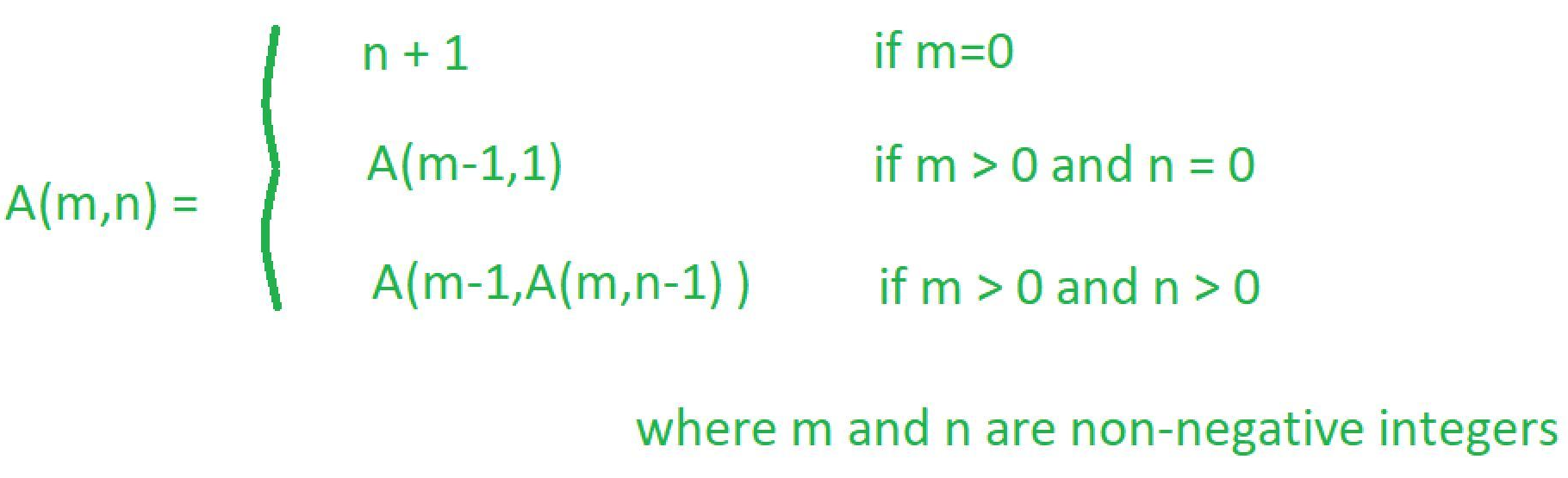Examples:

Input: M = 2, N = 2
Output: 7

Input: M = 2, N = 7
Output: 6141004759

The approach for Ackermann function described in this article, takes a very huge amount of time to compute the value for even small values of (M, N) or in most cases doesn’t result in anything.

Dynamic Programming approach:

Here are the following Ackermann equations that would be used to come up with efficient solution.

A(m, n) = A(m-1, A(m, n-1)) —– (Eq 1)

A(0, n) = n+1 —– (Eq 2)

A(m, 0) = A(m-1, 1) —– (Eq 3)

Let’s assume the value of m = 2 and n = 2

A 2d DP table of size ( (m+1) x (n+1) ) is created for storing the result of each sub-problem.

Following are the steps demonstrated to fill up the table.

1. Empty Table – Initial Step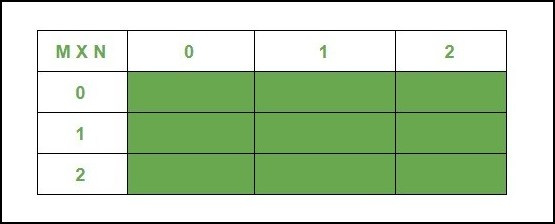2. Filled using A ( 0, n ) = n + 1
The very next method is to fill all the base values, by taking the help of equation-2.

3. In the next step the whole 1st row would be filled,

• A ( 1, 0 ) = A ( 0, 1 ) —– (refer Eq (3))
Since A ( 0, 1 ) = 2
Therefore, A ( 1, 0 ) = 2 —–(Eq 4)

• A ( 1, 1 ) = A ( 0, A ( 1, 0 ) ) —– refer Eq (1)
= A ( 0, 2 ) —– refer Eq (4)
= 3 —– refer Eq (2)
So, A ( 1, 1 ) = 3 —–(Eq 5)

• A ( 1, 2 ) = A ( 0, A ( 1, 1 ) ) —– refer equation (1)
= A ( 0, 3 ) —– refer equation (5)
= 4 —– refer equation (2)
So, A ( 1, 2 ) = 4 —–(Eq 6)

4. Fill the table using equations and stored values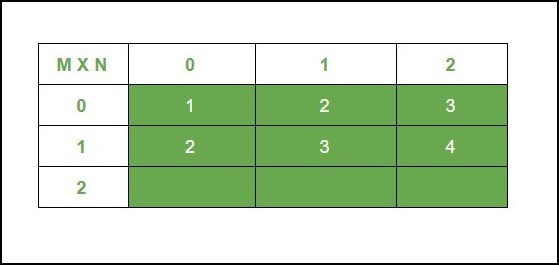Let’s just fill the first column of the last row i.e (2, 0) in the same manner as above, because for the other two columns there are some unsolved values.

A ( 2, 0 ) = A ( 1, 1 ) —– refer equation (3)
A ( 1, 1 ) = 3
So, A ( 2, 0 ) = 3 —– (Eq. 7)

5. Solving for A ( 2, 1 ) and A ( 2, 2 ).

For simplicity the process for solving above functions is divided into two steps,

• In the first one, the problem is identified.

A ( 2, 1 ) = A ( 1, A ( 2, 0 ) ) —– refer equation (1)
A ( 2, 0 ) = 3
A ( 2, 1 ) = A ( 1, 3 )

So to compute this value, again use equation (1)
A ( 1, 3 ) = A ( 0, A ( 1, 2 ) )
A ( 1, 2 ) = 4
A ( 1, 3 ) = A ( 0, 4 ) —– refer equation(2)
= 5

Therefore A ( 2, 1 ) = A ( 1, 3 ) = 5 — (Eq 7)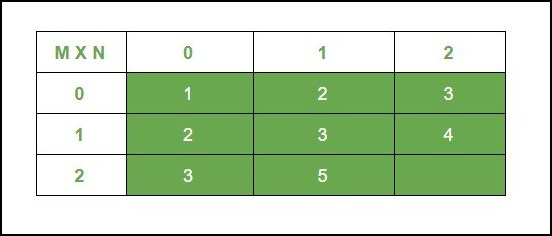• In the next one, methodology is described in detail and a generic formula is obtained to be logical while being used in program

Let’s solve A ( 2, 2 ) , with a theory upfront

A ( 2, 2 ) = A ( 1, A ( 2, 1 ) ) —– refer equation (1)
A ( 2, 1) = 5
A ( 2, 2 ) = A ( 1, 5 )

To compute A(1, 5) in a generic manner, observe how it reduces itself!

A ( 1, 5 ) = A ( 0, A ( 1, 4 ) )
A ( 1, 4 ) = A( 0, A ( 1, 3 ) )
A ( 1, 3 ) = A ( 0, A ( 1, 2 ) )
A ( 1, 2 ) = 4

Returning back from the function we get,
A ( 1, 3 ) = A ( 0, 4 ) = 5 —– refer equation (2)
A ( 1, 4 ) = A ( 0, A (1, 3 ) ) = A ( 0, 5 ) = 6 —–Since A ( 1, 3 ) = 5
A ( 1, 5 ) = A ( 0, A ( 1, 4 ) ) = A ( 0, 6 ) = 7
So, A ( 2, 2 ) = 7 ——- (Eq 9)

Important Points:

(n = column number, c: ( any number > n), r: row number]

1 . A ( 1, c ) = A ( 1, n ) + ( c – n ) From the Above Observation

2 . A ( r, c ) = A ( r, n ) + ( c – n )*r Based on hand tracing

6. Final Table with result of each sub-problem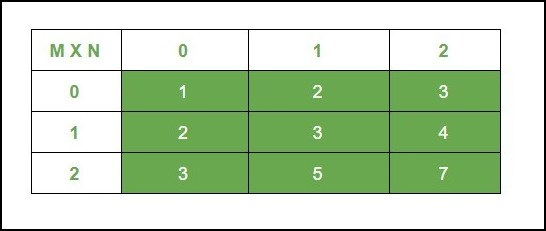Below is the implementation of the above approach:

## Python3

 `# Python code for the above approach ` ` `  `# Bottum Up Approach ` `def` `Ackermann(m, n): ` ` `  `    ``# creating 2D LIST ` `    ``cache ``=` `[[``0` `for` `i ``in` `range``(n ``+` `1``)] ``for` `j ``in` `range``(m ``+` `1``)] ` `    ``for` `rows ``in` `range``(m ``+` `1``): ` `        ``for` `cols ``in` `range``(n ``+` `1``): ` `            ``# base case A ( 0, n ) = n + 1 ` `            ``if` `rows ``=``=` `0``:        ` `                ``cache[rows][cols] ``=` `cols ``+` `1` `            ``# base case  A ( m, 0 ) =  ` `            ``# A ( m-1, 1) [Computed already] ` `            ``elif` `cols ``=``=` `0``: ` `                ``cache[rows][cols] ``=` `cache[rows``-``1``][``1``] ` `            ``else``: ` `                ``# if rows and cols > 0 ` `                ``# then applying A ( m, n ) =  ` `                ``# A ( m-1, A ( m, n-1 ) )  ` `                ``r ``=` `rows ``-` `1` `                ``c ``=` `cache[rows][cols``-``1``] ` `                ``# applying equation (2)  ` `                ``# here A ( 0, n ) = n + 1 ` `                ``if` `r ``=``=` `0``:     ` `                    ``ans ``=` `c ``+` `1` `                ``elif` `c <``=` `n: ` `                    ``# using stored value in cache ` `                    ``ans ``=` `cache[rows``-``1``][cache[rows][cols``-``1``]] ` `                ``else``: ` `                    ``# Using the Derived Formula  ` `                    ``# to compute mystery values in O(1) time ` `                    ``ans ``=` `(c``-``n)``*``(r) ``+` `cache[r][n] ` ` `  `                ``cache[rows][cols] ``=` `ans ` ` `  `    ``return` `cache[m][n] ` ` `  `# very small values ` `m ``=` `2`      `n ``=` `2` ` `  `# a bit higher value ` `m1 ``=` `5`       `n1 ``=` `7` ` `  ` `  `print``(``"Ackermann value for m = "``, m, ` `      ``" and n = "``, n, ``"is -> "``,  ` `      ``Ackermann(m, n)) ` ` `  `print``(``"Ackermann value for m = "``, m1,  ` `      ``" and n = "``, n1, ``"is -> "``,  ` `      ``Ackermann(m1, n1)) `

Output:

Ackermann value for m = 2 and n = 2 is -> 7
Ackermann value for m = 5 and n = 7 is -> 6141004759

Time complexity: O( M * N )
Auxiliary Space complexity: O( M * N )

Attention reader! Don’t stop learning now. Get hold of all the important DSA concepts with the DSA Self Paced Course at a student-friendly price and become industry ready.

My Personal Notes arrow_drop_upIf you like GeeksforGeeks and would like to contribute, you can also write an article using contribute.geeksforgeeks.org or mail your article to contribute@geeksforgeeks.org. See your article appearing on the GeeksforGeeks main page and help other Geeks.

Please Improve this article if you find anything incorrect by clicking on the "Improve Article" button below.